## 24        Mathematical Models

Taeke de Jong, R.P. de Graaf

24.1    Origins.................................................................... 2

24.2    The mathematical model is no reality...................... 2

24.3    Mathematics is the language of repetition.............. 3

24.4    Numbering(serial, sequence)................................. 3

24.5    Counting................................................................. 3

24.6    Values and variables............................................. 4

24.7    Combinatorics........................................................ 5

24.8    Taming combinatorial explosions........................... 7

24.9    The programme of a site........................................ 8

24.10   The resolution of a medium.................................... 9

24.11   The tolerance of production................................. 11

24.12   Nominal size systems.......................................... 12

24.13   Geometry............................................................. 18

24.14   Graphs................................................................. 20

24.15   Probability............................................................. 25

24.16   Linear Programming (LP)...................................... 27

24.17   Matrix calculation................................................. 29

24.18   The Simplex Method............................................. 30

24.19   Functions............................................................. 32

24.20   Fractals................................................................ 32

24.21   Differentiation....................................................... 33

24.22   Integration............................................................ 34

24.23   Differential equations........................................... 37

24.24   Systems modelling............................................... 38

A curriculum in mathematics for the Architecture Faculty at Delft University, taught for a few years during the nineties by the Mathematics Faculty, was ill-suited to the architecture staff, including its examples and references. So, the students who could not understand what use it was, experienced more nuisance than stimuli while designing, and were avoiding mathematics in the curriculum as a whole, where other disciplines could compensate for low grades in mathematics. The practice of design was doing well with high-school maths with some extensions, so why bother?

The realistic production of form is, as always, superior to the abstract mathematical detour via form description by Cartesian co-ordinates, even if fractal forms are generated. The mathematician and designer Alexander has been more successful with his ‘Pattern Language’  than with his ‘Notes on the Synthesis of Form’. The remainder of mechanics and construction physics is being taken care of by specialised consultant agencies and computers following delivery of a sketched design. No senior designer has any recollection of the content of mathematical education (s)he was exposed to during the sixties and seventies, when it was compulsory, while the practice featured nothing that might benefit from remembrance.

Of the lecture notes on architecture, ‘geometry’, ‘graph theory’, ‘transformations and symmetries’, ‘matrix calculation’ and ‘linear optimising’, ‘statistics’, ‘differential and integral calculation’ composed and, in the nineties, introduced in a simple form with the problem-orientated education only the latter may be found in the Faculty’s bookshop anno 2002. This last relict is due to the tenacity of the sector Physics of Construction. During the more mature years of building management matrix calculation for optimising exercises is being brushed-up from high-school maths. Then one is lacking the lost foundation in the first year. With the slow filtering-through of end-user friendly computer applications, as there are spreadsheets and CAD (pixel and vector presentations of form) during designing a new interest is dawning. Computer programs like Excel, MathCad, Maple or MatLab do ease experimenting with mathematical formulae as never before.

From these mathematical ingredients, also adop­ted by Broadbent as relevant to architectural design, Maybe a new mathematics for architecture might be composed. Architecture itself and civil engineering, it should be remembered, were standing at the cradle of mathematics. This Chapter does not pretend to stand at the birth of a new building mathesis. As urban architects, its authors fall short of the proper attainments. However, it gives a global survey of mathematical forms that may be employed in ar­chi­tectural design – with a reading list – providing linkage with a new element as a point of departure: combi­natorics. Whoever wants to brush up on high-school maths or to get a bird’s eye view of mathematics as a whole is referred to publications pertaining thereto. Experimenting with the Excel computer programme is especially recommended. In spite of that, Euclid’s answer to the question whether there would not be a simpler way to study geometry than his ‘Elements is still applicable: “There is no regal road to geometry’.

### 24.1       Origins

Mathematics is a language developed in order to describe locations, sizes (geometry), numbers (arithmetic) and developments from observations (measurements and counts), to process these descriptions and to predict new observations on that basis. In this vein, until 500 BC, for the founding of cities in Greek colonies a square of 50 x 50 plethra (a ‘plethron’ is some 30 metres) was paced off thanks to a diagonal of 70 plethra, in order to realise straight corners.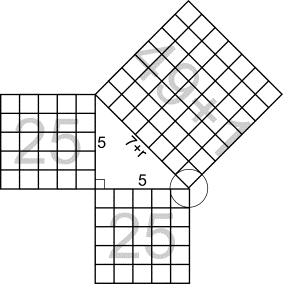Figure 1 Pythagoras

Pythagoras (580 – 500 BC) – or one of his pupils – next provided the well-known proof that the ratio should be slightly larger than 7 to 5; while the square of 7 is just one unit less than 25 + 25. From the womb of geometry, thus, the arithmetical insight was born that real numbers RR exist – such as the square root of 2, or the real length, derived therefrom, of the diagonal – not to be attained by simple partitioning (rational, QQ) of natural numbers (NN = 1, 2, 3 …). Geometry – literally: ‘measuring of the land’ – owns its first described development to the annual flooding of the river Nile. In ancient Egypt, floods wiped out the borders between estates and property, so that each year it had to be determined geometrically who owns what; as seen from standpoints which were not flooded. Arithmetic has roots in Phoenician trade. The Greek Euclid (around 300 BC) collected knowledge in both senses of his day and age in his book Elements, (via the Arab world) the cornerstone of all education in geometry well into the twentieth century. Euclid used earlier texts, but derived as the first the propositions of geometry by logical reasoning from 5 axioms. With this, a process was completed emancipating mathematics from empirical practice of measuring and counting examples.

### 24.2       The mathematical model is no reality

Since then, mathematics can, like logic, without observing the sizes of input (non-empirically, a priori) come to new forms of insight (synthetic judgements). Logical proof dominating, they are accepted as new insight also without observing sizes of output. The philosopher Kant (1724 – 1804) struggled with the question how that is possible at all: synthetic judgement a priori. For an empirical-scholarly theory always states that in the case of an independent observation from a set X, a corresponding independent observation from a set Y follows. If the elements of X and Y may be put in a corresponding, following, order so that they form the variable x and y, one may interpolate observations that have not been performed, while at constant conditions (ceteris paribus) – not included in the model – extrapolating to the future. A regularity of their correspondence is regarded as a working between them: y(x). Probable (causal) as well as possible (conditional) workings exist. If, for instance, the size of the population (x) is increasing, the number of buildings (y) is increasing as well following a hypothetical working y(x).

The larger the number of observations (n), the more convincing the theory. If one can demonstrate, by way of one hundred time sequences (n = 100) that between the two of them a working (function) may be defined that convinces more than one single correspondence in the past year (n = 1). At larger numbers of independent input observations, the scholar can look for a mathematical working y = f(x) (e.g. y = ½ x) producing the same results as dependent observing (modelling). A just input and output must show a perceptible relation to reality, not the mathematical operations employed by way of a model. Different (mathematical or real) workings (functions) may yield the same result. As soon as other facts are observed than those predicted, the empirical theory is rejected; not necessarily the mathematical discourse playing a rôle therein; although the two occasionally get confused. If the predictions are confirmed, in its turn, the mathematical working model is often regarded as ‘discovered’ reality (‘God always calculates’). However, this is not necessary in order to accept a theory (until the opposite has been proven).

### 24.3       Mathematics is the language of repetition

Just as in daily parlance, in logic and mathematics as well, concepts (statements, expressions) are used and composed into a model (declarations, sentences, full-sentence functions, workings, functions) with operators like verbs and conjunctions. Logic is using these operators particularly in the case of conjunctions (for instance: if P, then Q) mathematics the verbs (functions such as adding and summing). Logical deductions in mathematics usually have the logical linguistic form: ‘if working P, then working Q’. However daily parlance has the capacity to name unique performances. This primal declarative function of everyday language has the character of a contract. Only when the performance has been witnessed anew is there a rational ground to start counting. What is repetitive is food for mathematics. For all mathematical operating, name-giving, as in everyday language, is – usually implicitly – pre-supposed. However, mathematics is of no use in unique performances. If one stone weighs one kilogram, then two stones weigh only two kilograms if they are ‘equal’. This equality (here in terms of size and material) can only be agreed on by normal words. Sub-dividing dissimilar stones in equal fragments (transforming sizes in numbers, analysis) can make unique specimens elective for counting, and then for mathematical operations based on that counting. The question whether that can be done will always remain; as in Solomon’s judgement: two times half a child means no child anymore. In analysis a connectivity, incorporating the essence of the architectural object, may get lost and will remain lost during synthesis to a different magnitude (counting). The scale paradox may be a nuisance while sub-dividing an object again and again in increasingly smaller parts, in order to compose next from this a different order of magnitude or to predict it (infinitesimal calculation, differential and integral calculation). The other, lost properties – in a specific context – may be taken into account next in the formulae (increasing validation, see page ), but this is just shifting the problem.

### 24.4       Numbering(serial, sequence)

Numbering(serial)  just pre-supposes difference in place, not similarity in nature. I can enumerate the total of different objects in my room (or letter them) in order to be able to see later whether I am missing something, but this numbering(serial) does not allow mathematical operations. In spite of the fact that they are mathematically greatly important, since ordered difference of place (sequencing) is crucial in number theory. The number(serial) serves as a label, name, identification (identification number, ID number, or index, in the case of variables) that may prevent exchanging, missing and double counting. By the same token, it is impossible to calculate with these numbers, although ‘numbering(serial)’ is pre-supposed silently in the case of ‘counting’. Sequencing of numbers has in principle no other purpose than that it is staying the same, even if the numbered objects are changing later in place. The number stabilises differences in place ever witnessed as if on a photograph.

Nevertheless, the sequence, in which one is numbering(serial), often gets, in practice, a meaning (for instance: in the order of arrival), allowing conclusions. Although one is inclined to introduce with an eye on that some logic in a numbering (categorising), it is halted sooner or later, while numbering is incurring lapses or lack of space. At current capabilities of information processing, this is why it is advisable, for instance, to open in a spreadsheet a database next to the column with sequential identification numbers (always to be produced independent of the shifting row and column numbers!) new columns in order to distinguish categories on which one wants to sort. For the mathematics it is important that it is possible to number(serial) input and output numbers, to index, identify and retrieve from a database in a fixed sequence and combination. The number(serial) is the carrier of the difference of place in a database. A reliable database is carrier of differences in nature and place in the reality (not that nature and place itself). To ensure that an identification code will always be pointing at the same object it should be invariant during the existence of that object. Additionally it is not allowed to have meaning in terms of content, such as a postal code and house number combination, for this changes in the case of home-moving the corresponding ‘object’.

### 24.5       Counting

Observations can only be expressed mathematically if they are occurring more than once (in a comparable context) and may be harboured as ‘equal’ in any sense in a set. Only then can one count them. The equality pre-supposition of set theory and mathematics is sometimes forgotten, or all too readily dissolved, by analysis (changing scale by concerning smaller parts). In this way an area of 1000 m2 can be measured by counting, but each square metre has in many respects a different value that makes the area found in itself (without weighing) meaningless. The equality pre-supposition can lead to mathematical applications without sense, when the set described is too heterogeneous qua context or object for weighing. In this vein I can count the number of objects in my room, but each and every mathematical operation on this number alone does not lead to useable conclusions. Some objects are large, others small, some valuable, others not; or not elsewhere. From the number I might perhaps derive the number of operations in case of moving home, but these actions will be differing in their turn with the nature of the objects. Still I can say: “If I throw away something, I have less to move.” If I throw away a moving box with that argument, I have less to move, but this has no relation anymore with the effort (larger without the box) of moving that may have fostered the argument. Mathematical modelling would be misleading here and requires a comparable context.

So: some equality in nature is already pre-supposed when it comes to counting. Curious­ly enough, a difference is also pre-supposed: when counting, I am not allowed to point at the ‘same’ object twice (double counting). The objects pointed at should differ! What this difference in identity exactly is, is left here undecided; for reasons of convenience we call it ‘difference of place’, although this does not cover, for instance, the problems involved in counting moveable objects like butterflies on a shrub, mutually exchanging places. A number, or variable, therefore pre-supposes equality of nature and difference of place, even if that place is not always the same.

The equality in nature pre-supposed when it comes to counting does require the definition of a set (determining which objects we count or not). This definition is pre-supposing within the set defined equality, but at the same time to the outside difference with other sets. This paradox is explained elsewhere in the book as a ‘paradox of scale’ or ‘change in abstraction, see page . In addition the ‘nature’ is not possible to change during counting. It is not allowed to use one century for counting one basket of apples, since it is likely that after an age like that the apples will not exist anymore.

The difference of place pre-supposed at the moment of counting does require a unique indication of place (which objects were already dealt with or not yet). By the same token such an indication of place is pre-supposing distances between the places (intervals) or between their centres (core to core distance); if that would not apply they would not differ and be unique. The size of the places indicated (scale) should be equal to the one of the objects placed (extension); if that would not apply one place could contain several similar objects, pre-empting identification of the objects themselves. Rather paradoxically, difference of place (uniqueness) is also pre-supposing an equality of scale (unit or order of magnitude) in order to guarantee that uniqueness. An object without scale (a point) may still be identified by mutual intervals. If these are small enough, points may produce a line, a surface or a volume.

### 24.6       Values and variables

Therefore, we are counting by pointing at similar objects differing in their various places (in order to avoid double counting). Since that place may change, we make a snapshot, stating for the differences of place a randomly chosen, but fixed sequence, numbering(serial). To each indication a different name is given, number(serial). The final number is the number(quantity) or figure. Sometimes the sequence is of no importance, so that it is possible to restrict oneself to a uniquely identifying naming (nominal values). When the sequence imports, the values are termed ‘ordinal’. Next, when the intervals between the objects numbered are equal, we call the number an ‘integer’. This enables operations with interval-valuessuch as adding, subtracting, multiplicating and dividing, without the need to indicate, count or re-count the objects and their places. With this different counts may be predicted from certain counts, but the result may also be a ‘non-object’. By naming this outcome ‘zero’ according to a price-less discovery of the world of Islam, and even by extending it after boundless subtracting with ‘negative numbers’ calculating is not restricted to objects accidentally present.

This is opening the road to calculation without reference to existing objects. By taking zero for a point of departure with at both sides the same interval as between the other numbers, the distance of this zero point to two numbers can provide a relational number (rational value). This is the foundation for measuring. Sometimes this results in fractions, that may be expressed in ‘rational numbers’. If they are represented as points on a line of numbers, it becomes apparent that in between the numbers resulting from division of integral numbers still other values exist (real numbers). Ratios can also yield a relation between numbers of different kinds of objects (for instance inhabitants per residence: residential occupation). The set of values of one kind is called ‘variable’. Function theory tries to work out arithmic rules for predicting, from the development of one variable x, another variable y. It is often difficult to determine, whether x and y entertain also a causal relationship, or are just demonstrating some connection (correlation); for instance on the ground of a common cause, a third variable, or if a great many causes and conditions are at work.

Particularly in probability calculus chances and probability distinguishing between these various kinds of values is important. Then large numbers of results of a process are taken into consideration and the chance of a few of them is calculated (event). Extreme values are occurring less often as an outcome of many natural processes than values in-between. As a measure for the distribution between the extreme outcomes the average and its mean, the median and the modus are used. The average of a large number of values can only relate to interval values or rational values. In the case of ordinal values, no average exists, but a median (as many outcomes with a higher as with a lower value). In the case of nominal values it is also impossible to calculate a median; then a modus can be used (the number of values occurring most frequently).

When a set of values X is now compared to a different set Y in order to find between both a correlation, various statistical arithmetic methods (tests) exist, depending on the kind of values representing X and Y:

 Y X Nominaal Ordinaal Interval & rationaal Nominaal (dichotoom) Kruistabel Mann-Whitney ·          Grote steekproef: t-toets/ANOVA ·          Kleine steekproef, Y normaal verdeeld: t-toets/ANOVA ·          Kleine steekproef, Y niet normaal verdeeld: Mann-Whitney Nominaal (niet di-chotoom) Kruistabel Kruskal-Wallis ·          Grote steekproef: ANOVA ·          Kleine steekproef, Y normaal verdeeld: ANOVA ·          Kleine steekproef, Y niet normaal verdeeld: Kruskal-Wallis Ordinaal n.v.t. Rang-correlatie ·          Rangcorrelatie Interval & rationaal n.v.t. n.v.t. ·          Grote steekproef: Pearson-correlatie ·          Kleine steekproef, X en Y normaal verdeeld: Pearson correlatie ·          Kleine steekproef, X en Y niet normaal verdeeld: rangcorrelatie Table 1 Overzicht toetsen op paarsgewijze samenhangen tussen twee kansvariabelen X en Y

In this the nominal values have been distinguished in dichotomous (yes – no) or non-dichotomous (multi-valueous).

### 24.7       Combinatorics

As soon as counting has been mastered, one may name on a higher level of abstraction the internal categories (k), pre-supposed to be homogeneous, and unique places (n) themselves; number and count them. Allocating over the available places the kinds and within it the number of kindred cases (p) is the subject of combinatorics. It is pre-supposed in numerical systems and, therefore, a fundamental root of mathematics. This way the number of possible arrangements of 10 names over 2 places equals 100, over 3 it is 1000. Due to the Islamic discovery the notation of large numbers by combination of cipher names has become simple and more accessible to calculations.

Combinatorics may also be regarded as a basic science for architectural designing. More generally, one may calculate the number of possible arrangements, without any restriction, of k categories over n niches as kn. When it is supposed that 100 different kinds of building materials (among them air, space) may be used on a site of 100m2, with 1 mln inter,connecting allocation possibilities of 10 x 10 cm, this is yielding already in a flat surface many more design possibilities (1001000000) than there are atoms in the universe (10110). The designer is travelling, so to speak, in a multiple universe of possibilities, where the chance of meeting a known design is practically nil. With this, to all practical purposes, infinite number of possibilities no rational choice is possible by taking them all into account. Also, when restricted by a programme of requirements, in which the units ‘space’ must be positioned in certain amounts in inter-connection, the number of possibilities is still practically infinite. The various mathematical disciplines passing muster in the following paragraphs as possibly relevant for architecture, are described there as rational restrictions of this number of possibilities.

The designer, faced by a white sheet of paper or a blank screen, is asked to indicate on it difference in place (state of dispersion; form) and in kind (colour). The differences in nature are contained in a range to be generated (the 'legend', in its original connotation) spread in k units of colour (e.g. the programme) over n niches (appropriate fields, for instance on the grounds of the site); the differences in place.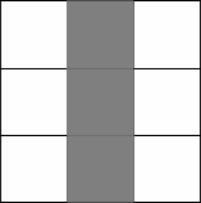n = 9 k=3, scheme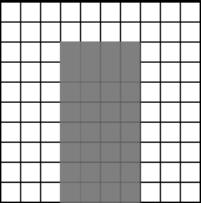n = 100 k=32, rough draft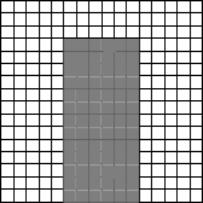n = 256 k=78, Windows-icon 2 A programme, approx. 1/3 of the site, spread over the ground in 3 resolutions.

How many different states of dispersion can be generated in total? In mathematics the branch of combinatorics deals with that kind of 'arrangements' in finite sets and with counting the possibilities of arrangement under suitable conditions.

If a range of k = 3 colours is available for colouring n = 3 fields, 33 = 27 variations apply. In this way the colours red, white and blue combined with three fields yield 27 distinct flags.

More generally: V(n,k) = kn (variations­ with repetition). With as many colours as niches the expression reads nn.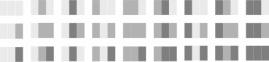aaa aba aca baa bba bca caa cba cca aab abb acb bab bbb bcb cab cbb ccb aac abc acc bac bbc bcc cac cbc ccc Figure 3 V(3,3) = 33 = 27 variations

Among them, however, there are many cases in which colours have been repeated or omitted. True 'tricolores' are but few. The first condition to be added amounts to the presence of all three colours. Colour one has 3 positions available; for the second 2 remain; and for the third 1. This limits the number of cases to 3 x 2 x 1 = 6, abbreviated to 3!, so-called permutations. More generally, one may write: P(n) = n! (permutations without repetition).

 niches n just as much different colours as  niches 1 a 2 ab ba 3 abc acb bac bca cab cba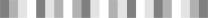Figure 4 P(n) = n! : 1, 2 en 6 permutations

### 24.8       Taming combinatorial explosions

Permutations restrict the ultimate number of variations: 3!, is less than 33; n! rises less fast than nn, but faster than, for instance nn/2, when only half the amount of niches is used for the number of colours ‘Factorials limit the largest powers’. Yet, permutations do increase fast enough to lead to a 'combinatorial explosion' on higher values for n: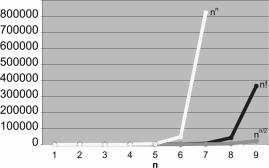Figure 6 Combinatorial explosions

How to select from all these possibilities? To many people this is a crucial question in personal life and in designing. With so many possibilities a conscious choice does not apply actually, so that during the reduction of the remaining possibilities, still not yet imaginable, one is guided by contingency, emotion of the moment, sensitivity for fashion, or routine. Deelder says: ‘Within the patches the number of possibilities equals those outside them.”; as long as the resolution is lowered.

Although mathematics is used generally in order to arrive at singular solutions, where different possibilities are not taken into account to the dismay of the designer, this discipline may also be used to reduce the remaining possibilities within exactly formulated, but in other respects freely varying conditions (for instance a scale system of 30 x 30 x 30 cm) and to survey them.

The branches of mathematics relevant to architecture are therefore seen here as the formulating of these restrictions on the total amount of possible combinations.

Systems of measure are mathematical sequences reducing the sizes of components to convenient sizes.

Geometry restricts itself to connected (contiguous) states of dispersion (shapes) such as lines, planes, contents, which can be described with a few points and with a minimum of information as to their edges.

Graph theory restricts itself to the connections between these points (lines with or without a direction) regardless of their real position.

Topology formulates transformations of forms and surfaces, so that one form can be translated in another one by a formula. This involves the direct vicinity of each point in the set of points determining this surface. For minimising the curved surfaces between the closed curves (soap skins) the forgotten mathematical discipline of the calculation of variances is necessary. This mathematics is also applied to the tent roof constructions of Frei Otto. However, this discipline is too complicated to be introduced in this context.

Probability theory and its application in statistics restricts itself to the occurrence of well-defined events, for instance the happening of one or more cases among the possible cases in a given space or time.

Optimising by linear programming aided by matrix calculations formulates the remaining exactly restricted possibilities as the 'solution space’.

Next to these mathematical restrictions there are any number of intuitive restrictions causing the disregard of possibilities. If one recognises, for instance, in the illustrations on page 6 and following the image type of a door in a wall, the basis of the door (programme k) should lie in the lower part of the wall (the space present n), unless a staircase is drawn as well.

### 24.9       The programme of a site

With variations (kn) and factorials or permutations (n!) alone it is impossible to determine the number of cases when a particular colour has to be present in a predictable amount (a quantitative 'programme' per colour). With a programme for an available space n, where one colour (for instance black) has always to be present at least p times P(p,k) = n!/p! possibilities exist (permutations with repetition). The p! in the denominator of the ratio restricts the explosive effect of n! in the numerator at higher values of n, except of course, if it equals 1. That applies in the first column of the figure below; if p = 1 (so p! = 1) it has no effect on the ratio; the number of permutations P remains, as in the previous example with letters, 4! = 24. At p = 2 (p! = 2) and p = 3 (p! = 6) the number is restricted.

 P(4,1) = 4!/1! = 24 P(4,2) = 4!/2! = 12 P(4,3) = 4!/3! = 4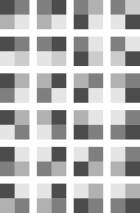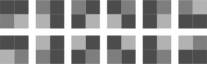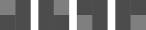Figure 7 Permutations in 4 niches, with at least k = {1,2,3} black elements combined with other hues.

The larger the programme p of one colour (in this case black) with regard to the total space available, the less possibilities remain for other colours to generate cases. Evidently, p, the number of colours surfaces to be distributed may not exceed the space n available.

The formula pre-supposes that the niches remaining will be filled with as many different colours. They generate the additional cases, not requiring attention from a programmatic point of view.

If not only the programme p, but also the complementary remainder n - p is combined into one colour, no other colours remain to generate additional cases. A permutation with two colours, p1 and p2 equals the formula n! / p1!p2! The possible arrangements of programme p1 and the remainder p2 develops into n! / p!(n-p)! (Newtons binomium).

Within both surfaces, the sequence in which the niches are filled in with one colour is now irrelevant. The only consideration of importance left is the number of different instances in which one quantity p is allotted to n possibilities without a rôle for its own sequencing. This entity plays a leading part in statistics. Factor p then is the number of possibilities of an event, n – p is its complement of possibly not happening that event. So, not only possibilities in space apply: n may also reflect time. A little counter-intuitively the common expression is 'combinations'; and one simplifies the formula P (n, p1, p2 ) = n! / p1! p2! to C (n,p) = n! / p! (n - p)! ; or shorter still: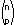, 'n over p'.

(combinations without repetition).

 C(4,1) C(4,2) C(4,3) 1x2x3x4/ ((1x)(1x2x3)) = 4 1x2x3x4/ ((1x2)(1x2)) = 6 1x2x3x4/ ((1x2x3)(1)) = 4 abbb,babb, bbab,bbba aabb,abab,abba, baab,baba,bbaa aaab, aaba, abaa, baaa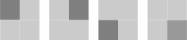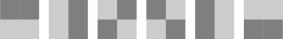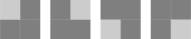Figure 8 Combinations in 4 niches of 2 colours

Without fail, one will experience the highest level of freedom of design if a one-colour programme occupies half the site available (p1 = p2); a sound reason to plead with the principal for as much open space as space built.

At higher resolutions as shown in the diagrams on page 6 even the number of black-white combinations rises again explosively C(9,3) = 84, C(100,32) = 140 000 000 000 000 000 000 000 000 (1,4E+26 for short in Excel), the Windows-icon shown (16pixels x 16pixels = ­256pixels), amounts at 78 black pixels C(256,78) already to 1,2149E+67 images. Excel calculates this with the command = COMBIN(256,78).

Again, the greatest freedom to design is found in an equal distribution between black and white pixels: C(256,128) = 3,50456E+75. If 256 colours can be used the variance formula is 256256 (10616 or 22048). This exceeds the number of atoms in the universe by far (10110 or 2365).

If one studies, for instance, 5625 locations of 1 km2 in the colours 'built' (815) and 'open' (4818), approximately 101008 or 23349 alternatives exist for the present 'Randstad' area in the Netherlands. The average PC cannot deal with this. Excel digests in this case at great pains p=156.

In this sense the designer is travelling in a multiple universe of possible forms (states of dispersion). The chance one runs into a known form is, in this combinatorical explosion, negligible

The number of elements of the programme (legends units, colours, letters) may be raised above 2; within the total sum of n, of course.

This enables the calculation of the numbers of cases belonging to a specified programme on a location. If one wants to allocate for instance 4 programmatic elements to n fields, there are

P(n,p1,p2,p3,p4) = n!/p1!p2!p3!p4! design possibilities. The total of the colour surfaces p1+p2+ … desired is again not allowed to exceed, of course, the surface available in total n. With a computer programme such as Excel one can calculate, for instance, that the possibilities of allocating 4 types of usage of 25m2 each to an area of 100 m2 is 2,5E+82.

### 24.10    The resolution of a medium

A pen, pencil or ball-point draws points and lines occupying a minimal surface, corresponding with the thickness of the material. Surfaces may then be depicted by filling in the surface with such lines. A computer screen is building an image from tiny surfaces (picture elements, pixels) that suggest contiguous lines or surfaces. This resembles the difference in the history of art between the Florentine (line-orientated) and the Venetian (point-orientated) ‘disegna’. A usual screen features 1024 x 768 pixels.

A pixel-orientated program (paint- or photo­program) just supports the colouring of these points like in painting. If a ‘line’ or ‘surface’ is over-written, the only way to restore it is to fill in the previous colour of the over-written pixels. More often than not foreground and background are not distinguished in layers allowing display layer by layer, or in combination.

A computer drawing program (vector program, drawing program or CAD) just takes together the essential points of a drawing into a matrix of co-ordinates and translates the drawing into pixels between these points as soon as it is activated. The pixels in between are not being remembered during storage, so that mass-storage space requires less space than a pixel image. In the case of an enlargement of the drawing or of a detail, the resolution adapts itself automatically, so that the lines are not blurred.

A vector is a matrix with one column (or row) that in the flat plane just needs two co-ordinates to be defined from an origin. In the figure below three vectors a, b, and b-a have been drawn. They illustrate the calculation rules that are of great service in drawing programs and applied mechanics.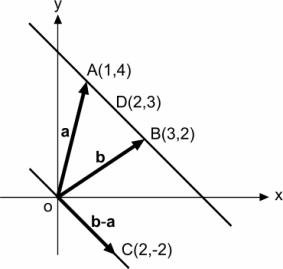Figure 9 Defining line segments by vectors

The line segment AB is represented by the co-ordinates of the vectors a and b; the points in between are calculated by giving a co-efficient I between 0 and 1 a sequence of values provided by the formula l (b-a)+ a.

 vector substraction l =0,5 A b b-a l(b-a) l(b-a)+a 1 3 2 1 2 4 2 -2 -1 3 Table 2 vector substraction and multiplication

This way I=0,5 yields the point in between D(2,3). The larger the number of values calculated, the greater the resolution of the line segment AB.

The combinatoric explosion of possibilities is already drastically reduced during the initial stage of the design by the coarseness or, in reverse, the resolution of the drawing. That is something different than the scale of a drawing.

The position of a deliberately coarsely sketched line must be judged according to commonly understood conventions within certain margins. The size of such a margin surrounding a drawn point we call ‘grain’. We call the radius R of the circle inscribed in the drawing as a whole ‘frame’, and the ratio of the radius r of the grain to R ‘resolving capacity’, or ‘resolution’. The ‘toleran­ce convention’ could be interpreted as ‘any sket­ched point may be interpreted within a radius r, by that interpretation transforming the rest of the drawing accordingly’. The tolerance of the drawing is expressed by r.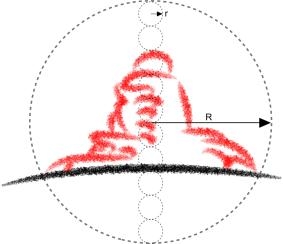Figure 10 The radius r of a grain here is approximately 10% of the radius R of the frame.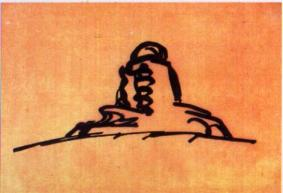Figure 11 Sketch approx. 10% resolution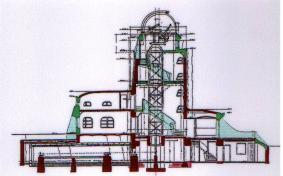Figure 12 Drawing approx. 1% resolution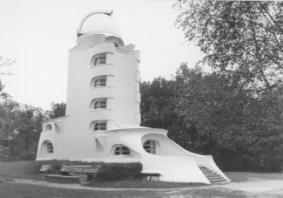Screen approx. 0,1% resolution source: http://aipsoe.aip.de/galerie/ausstellung/bilder Figure 13 Mendelsohn (1920) Einsteinturm (Potsdam)

A sketch with a grain of roughly 10% of the frame is known as a loose sketch. It is used in an early concept, a type or a schema. It is often produced with a felt-tipped pen; with the same order of magnitude as the grain of the drawing, by that means stressing the tolerance convention. A ‘design’ hardly has a smaller resolution than 1%. A blue-print or computer screen does not exceed 0,1%. Only at this level things like details in the woodwork of a door and its frame in a wall are displayed. The total concept of a work of architecture is highly influenced by details, observed by the zooming eye of an approaching user.

### 24.11    The tolerance of production

A door should be slightly smaller than its frame in order to acquire a functional fit. In addition a carpenter or machine makes frames and doors respectively slightly larger, or smaller, than the nominal size written on the blue print.

This is also taming the mathematical use of positions behind the comma for architecture and technique in general. One is not allowed to think anymore in terms of numbers representing a point on the line of numbers: they are representing a margin, a band-with, a distance or class on that line. The nominal size is indicated on the blue-print, but it is certain that this precise size will never be delivered.

The frame should be equal or larger than the nominal size, the door should be smaller, but how much? The limits put to this product tolerance must be calculated by weighing the price of the precision and the performance of the door as a closure. If the tolerance of the frame opening is 0 to + 1mm, and the tolerance of the door –1 to –2 mm, the crack-width will be 1 to 3 mm, divided over two sides.

In order to decide whether to accept a batch of doors and frames or to send them back, no absolute measures are taken into account, but rather margins, classes such as ‘too small’, ‘small’, ‘large’ or ‘too large’. They may vary within limits of tolerance. In the case of the frames of the example in the above these limits are vis-à-vis the nominal size M:

too small  < M + 0mm < ‘small’ < M + 0.5 mm < ‘large’ < M + 1mm < ‘too large’,

while for the doors the nominal measure

too small’< M –2 < ‘small’ < M – 1,5 < ‘large’ < M – 1mm < ‘too large’.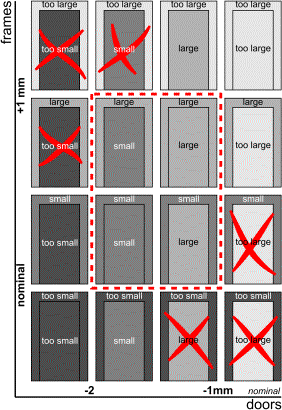Figure 14 Acceptible and not acceptible sizes

In first instance one assumes that they are not too small, nor too large (zero-hypothesis, dotted contour in the drawing) while a few are measured in order to see whether they are belonging to these classes yes or no. However on the basis of a random selection one can refuse the batch frames and doors on false grounds (fault of the first kind) or accept them on false grounds (fault of the second kind). The first fault is a producer’s risk, the second fault consumer’s risk. Since two different risks apply, both faults can not be minimised by taking the minimum of their sum. For instance the consumer’s risk may be systematically smaller than what one may derive from the second fault. If the producer, for instance, is delivering systematically too large or too small frames and doors, one may settle for a smaller refusal. If both are within reasonable margins (too) large, they will still have an acceptable fit and width of the crack (above right in the following figure). This also applies for a systematic deviation to the smaller side (below left). Only when the frame is (too) small and the door at the same time (too) large (below right), or vice-versa (above left), does the sizing cease to be acceptable.

### 24.12    Nominal size systems

A restriction to multiples of 30 cm (little less than a foot) is a well-known bridle to sizes in construction of buildings. It reduces the combinatoric explosion of design possibilities. A grid like that is used to localise foundations, columns and walls without design efforts for smaller sizes. A grid may have a different size in any one of the three dimensions. A preceding analysis of usage may yield an appropriate size of the grid for a specific function. The distinct multiples of the size of the grid yield distinct functional possibilities. In the preceding paragraph a grid is implicitly used.

An arithmatical series has an initial term a, a reason v and a length n. The terms increase along a straight line by steps of v, starting with a. An example is the height of the first floor a of a building and its successors with a height of v, resulting in the series of heights h (normal dwelling and flat building, a=v0):

 Normal dwelling Flat building Van Tijen (1932) n v h v h 9 2.85 27.15 8 2.85 24.30 7 2.85 21.45 6 2.85 18.60 5 2.85 15.75 4 2.85 12.90 3 2.85 10.05 2 2.6 8.0 2.85 7.20 1 2.6 5.4 2.85 4.35 0 2.8 2.8 2.70 1.50 -1.20 Table 3 Arithmical series in building

Another example concerns a building with one oblique wall, like the KPN building by Renzo Piano in Rotterdam. The oblique wall commences on first floor level. The initial term is representing here the fixed surface per floor (supposed to be 100 m2). The n-th term indicates the surface at the level of the n-th floor. The sum of the terms is the total surface of floors at the oblique wall.

 length starting term reason array sum n a v a+v*n 9 190 1450 8 180 1260 7 170 1080 6 160 910 5 150 750 4 140 600 3 130 460 2 120 330 1 110 210 0 100 10 100 100 Table 4 Arithmical sequence with sum

In these examples the reason remains equal, in the next example the reason changes each next n.

A sequence well-known from architecture and other arts is Fibonacci’s sequence: a new term equals the sum of two previous terms.

 length starting term reason array ratio n a v a+v*n r 9 3,4 8,9 1,62 8 2,1 5,5 1,62 7 1,3 3,4 1,62 6 0,8 2,1 1,62 5 0,5 1,3 1,63 4 0,3 0,8 1,60 3 0,2 0,5 1,67 2 0,1 0,3 1,50 1 0,1 0,2 2,00 0 0,1 0 0,1 1,00 0 0,1 Table 5 Fibonacci’s sequence

The reason v is variable now, but the ratio r between two adjacent terms converges at last to the ‘Golden Rule’, ‘Golden Section’ or ‘Divine Proportion’: the smaller number (minor m) than has a fixed ratio to the larger number (Magior M). The Magior M, again has the same ratio to the sum of both:

m : M = M : (m + M).

 From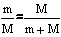follows: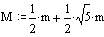For m = 1 follows for M/m and m/M: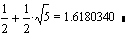and: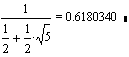In the case of a geometrical series the ratio r is a factor of multiplication, so that the ratio of two adjoining terms is a constant.

 length starting term ratio array n a r a*rn 9 2,000 51,20 8 2,000 25,60 7 2,000 12,80 6 2,000 6,40 5 2,000 3,20 4 2,000 1,60 3 2,000 0,80 2 2,000 0,40 1 2,000 0,20 0 0,1 2,000 0,10 -1 2,000 0,05 -2 2,000 0,03 -3 2,000 0,01 Table 6 Geometrical sequence

A geometrical series can be continued for negative values of n while the array remains positive for real architectural purposes.

Applications of geometrical series are found in the financial world, like in compounded interest and in annuities. An investment of € 1000,- at an interest of 5% yields after 1 year € 1000 * 1.05 = € 1050. After two years this is € 1050 * 1.05 or € 1000 * 1.05 * 1.05 = € 1102.50.

Experiencing sound is also an example of the geometrical series. An increase of sound to the amount of 10 dB is experienced as twice as loud; an increase of 20 dB as four times.

For architectural applications most geometrical series are not very useful, because adding architectural elements next to eachother (juxtaposition) produces new sizes, not recognisable anywhere else in the series.

However, when we choose the Golden Section as a ratio we get:

 length starting term ratio array n a r a*rn 9 1,618 7,60 8 1,618 4,70 7 1,618 2,90 6 1,618 1,79 5 1,618 1,11 4 1,618 0,69 3 1,618 0,42 ▲ 2 1,618 0,26 m+M 1 1,618 0,16 M 0 0,1 1,618 0,10 m -1 1,618 0,06 M-m -2 1,618 0,04 ▼ -3 1,618 0,02 -4 1,618 0,01 -5 1,618 0,01 Table 7 Golden Section

Now, every adjacent pair of sizes is flanked by their sum and difference, just as in the non geometrical Fibonacci sequence. Adding and subtracting of adjacent terms do not produce new sizes.

The differences in use of both proportion rules are only visible in the smallest stages: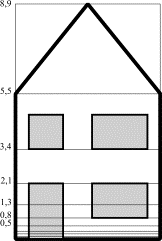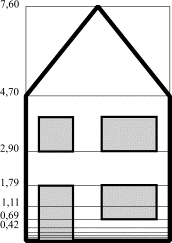Figure 15 Fibonacci house & Golden Section house

Many attempts have been made to recognise the Golden Section in nature and the human body. Until 1947 Le Corbusier took the human length of 1.75 m as a point of departure. Later, he started at 182.9 (red array) and 2.16 m (blue array): the reach of a man with a hand raised as high as possible.

 < 1947 Red array Blue array 0,2 0,2 0,3 0,3 0,4 0,4 0,5 0,6 0,7 0,9 0,9 1,1 1,4 1,5 1,8 2,3 2,4 3,0 3,7 3,9 4,8 6,0 6,3 7,8 9,8 10,2 12,6 15,8 16,5 Modulor 20,4 25,5 26,7 27 33,0 41,3 43,2 43 53,4 66,8 69,9 70 86 86,3 108,2 113,0 113 140 139,7 175,0 182,9 183 226 226,0 283,2 295,9 365,7 458,2 478,8 591,7 741,3 774,7 957,4 1199,5 1253,5 1549,0 1940,8 2028,2 2506,4 3140,2 3281,6 4055,4 5081,0 5309,8 6561,8 8221,3 8591,5 10617,2 13302,3 13901,3 17179,0 Table 8 Measure systems of Le Corbusier

The red and the blue columns are featuring steps with a stride too wide between the terms for architectonic application. For this reason, Le Corbusier combined them and rounded them off in the Modulor. There are many other attempts in making the possibilities of the design with scale sequences easy to survey and well to maintain in the design decisions with a pre-supposed, built-in beauty.

The problem of too large steps with the Golden Section was later solved by Reverend van der Laan. He selected the ‘plastic number’ r = 1,3247180 for a ratio. This is a solution of the equation r1 + r0 = r3 as well as of the equation r1 – r0 = rr-4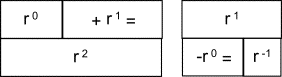Figure 16 Golden Section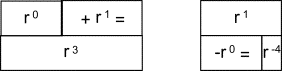Figure 17 Plastic Number

Since any number may be substituted for the initial term a, for instance another term from the series, the more general formulae rn+1+rn=rn+3 and rn+1-rn=rn-4 may be employed. In the row below this means that the formulae can be shifted, while keeping the mutual distance constant.

 length starting term ratio array n a r a*r^n 9 1,325 1,26 8 1,325 0,95 7 1,325 0,72 6 1,325 0,54 5 1,325 0,41 4 1,325 0,31 s 3 1,325 0,23 rn+rn+1=rn+3 2 1,325 0,18 1 1,325 0,13 rn+1 0 0,1 1,325 0,10 rn -1 1,325 0,08 -2 1,325 0,06 -3 1,325 0,04 -4 1,325 0,03 rn+1-rn=rn-4 -5 1,325 0,02 t Table 9 The plastic number

The sum and the difference between two consecutive terms are returning in the series as at the Golden Section, albeit 2 places upward or 4 places downward, rather than by 1 each time. Therefore they are forming with addition and subtraction no new measures while preserving the ratio r.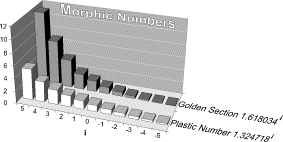Figure 18 Morphic Numbers

Aarts et al. have demonstrated that this ratio is, next to the Golden Section, the only one with this property. Together they are called ‘morphic numbers’.

### 24.13    Geometry

It is impossible to imagine a door distributed in tiny slits across a wall. Geometry restricts itself to contingent states of dispersion (lines, surfaces, contents) that can be enveloped by a few points, distances and directions. This pre-supposition of continuousness lowers the amount of combinatorial possibilities dramatically. The extent to which this geometrical point of view restricts the combinatorial explosion, is the subject of combinatorial geometry: it studies distributions of surfaces and the way these are packed.

However, the often implicit requirement that points in one plane should lie contingent within one, two or three dimensions is more obvious and self-evident in the case of a door, than in the one of a city. That is the reason why urban design is interested in non-contingent states of dispersion. The possibilities are restricted geometrically, following the often implicit pre-supposition of rectangularity. Particularly efficient production suggests this pre-supposition.

When one limits oneself to enclosed surfaces or enclosed spaces and masses, three simple shapes may  be imagined in a flat plane: square, triangle and circle. Why are they so simple? They survive as geometrical archetypes in geometry and construction everywhere.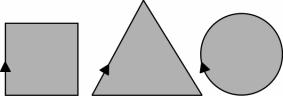Minimal number of directions in one loop Minimal number of changes of direction in one loop Minimal variation of changes of direction in one loop Figure 19 Simple shapes

Their simplicity may be explained by the minima  added in words to the diagram. This gives at the same time a technical motivation for application. A minimal number of directions is for production - e.g. sawing and size management - an effective restriction. Any deviation influences directly the price of the product. A minimal number of directional changes (nodes) is constructively effective (also from a viewpoint of stiffness of form). A minimal variation in directional changes(one without interruption, smooth) is effective with motions in usage; when one keeps the steering wheel of a car in the same position, a circle is described at last. They have been drawn in the diagram, with an equally sized area (programme). Their circumferences then are roughly proportioned like 8 : 9 : 7.

Our intuition meets its demasqué, by keeping the area equal: the triangle wins out. This visual illusion may be used for spaces needing particularly spatial power.

A second example of the capacity of geometry to lead to counter-intuitive conclusions about areas is the seeming difference in surface between the centre and the periphery as Tummers emphasises. In the diagram below they equal one another.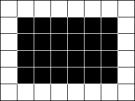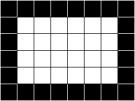Figure 20 Apparent difference in surface between centre and periphery

Functions characterised by the importance of inter-connectedness at all sides - like greenery, parks - tend to be better localised centrally, while differently structured functions (e.g. buildings) are better placed peripherally.

The triangle is playing an important rôle in geometry because all kinds of shapes on flat or curved surfaces and stereometrical objects may be thought of as being composed out of triangles. Measuring the surface, also on bent surfaces (geo-metry), is dependent on insight in the properties of triangles (triangulation) since it establishes a one-to-one relationship between lines and angles (trigonometry). In the measuring of angles (goniometry) next to the triangle the circle plays a crucial rôle. Descriptive geometry makes images of three-dimensional objects on a two-dimensional surface (projections), so that they may be reconstructed eventually on a different scale. With this, descriptive geometry is a fundamental discipline for architecture and technical design in general; for this description enables in its turn a wealth of mathematical and designing operations. The triangle also plays an important rôle in the technique of projecting.

These subjects have already been described thoroughly and systematically by Euclid in his ‘Elements around 310 BC. Until in the twentieth century this book has been the basis for education in ‘Euclidean geometry’. The work is available on the internet with interactive images and it is still providing a sound introduction into elementary geometry, as always. Together with the co-ordinate system of Descartes geometrical elements became better accessible to tools from algebra such as vectors and matrices (analytical geometry).

When objects can be derived with rules of calculation in a different way than by congruency, equal shape or projection, when they can be represented or shaped into another, ‘topology’ is the word. The properties remaining constant under these transformations – or oppositely change into sets of points – can be described along the lines of set theory or with algebraic means. This is leading to several branches of topology. What is happening during a design process, from the first concept via typing to design, is akin to topological deformation, but it is at the same time so difficult to describe, that topology is not yet capable of handling.

On the other hand, the existing topology is already an exacting discipline, pre-supposing knowledge of various other branches of mathematics, before the designer may harvest its fruits. Nevertheless, it is conceivable that a simple topology can be developed, restricting the combinatorical explosion of design possibilities rationally in a well-argued way, in order to generate surprising shapes within these boundary conditions. It would have to describe constant and changing properties of spaces, masses, surfaces and their openings in such a way, that complex architectural designs could be transformed in one another via rules of calculation. With this, architectural typology and the study by transforming design would be equipped with an interesting tool. The computer will play a crucial rôle in this.

When a design problem can be described in dimension-less nodes and connecting lines between them, graph theory could be a predecessor.

### 24.14    Graphs

When one notes in a figure only the number of intersections (nodes) and the number of mutual connections per intersection (valence, degree of the node) we deal with a graph.

A graph G is a set ‘connecting points’ (nodes, points, vertices) and a set of ‘lines’, branches (arcs, links, edges), connecting some pairs of connecting points together. A branch between node i and node j is noted as arc (i,j), shortened arci j.

Length, position and shape of the connecting points and branches are without importance in this. (In architecture the relative position of the connecting points vis-à-vis one another will probably be of importance.)

Among many figures corresponding types may be discerned wherein neither length nor area play a rôle (e.g. designing structure, not yet form and size). This enables the study of formal, technical and programmatical properties in space and time even before the sizes of the space or the duration in time are known.

A cube may serve as an example. It has 8 nodes. Each of them has 3 connections. This fixes the number of connecting lines (branches) : 8 X 3/2 = 12. For each connecting branch occupies 2 of the 8 x 3 connections in total.

 input output figure nodes connections per intersection (valence, degree) branches = nodes x valence/2 planes = branches - nodes+ 2 connections = branches x 2 boundaries = branches x 2 boundaries per plane (valence) = boundaries / planes tetrahedron 4 3 6 4 12 12 3 cube 8 3 12 6 24 24 4 octahedron 6 4 12 8 24 24 3 K3,3 6 3 9 5 18 18 3,6 K5 5 4 10 7 20 20 2,9 Table 10 Nodes and connections in regular solids

Following the formula of Euler, the number of planes = number of branches - number of nodes + 2. As soon as the planes (still without dimensions) come into the picture, we deal with a map. By the same token it suffices to count in a figure the intersections and their valencies in order to be able to calculate the number of branches and planes in the map.

The regular solids can be represented in a plane by a graph.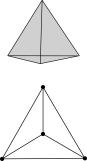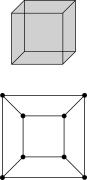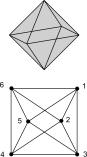tetrahedron cube octahedron 21 Regular solids as a graph

Based on this, the terminology of graph theory is readily explained. These are single graphs: there are no cycles arriving at the same node as the one of departure; or multiple connections between two nodes.

Furthermore, they are regular: in each node the number of connections per graph is equal.

The tetrahedron has a complete graph (K) unlike the other two, where possible connections - the diagonals - fail.

The graph of the cube clearly demonstrates that the outer area should be in calculated in order to count 6 planes. It is as if the cube is 'cut open' in one plane, in order to 'ex-plane' it on the page. It is immaterial which plane serves as outer plane. Graph theory does not yet distinguish between inside and outside.

The graphs of the tetrahedron and the cube are 'planar': the flatland does not feature crossings without intersection. The diagram below shows to the left an 'isomorph graph' for the octahedron where the number of nodes equals the number of valencies.

Compare the octahedron graph with the one of Figure 21.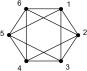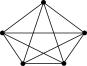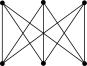octahedron K5 K3, 3 Figure 22 Octahedron, K5, K3,3

The branch between nodes 6 and 1 of the octahedron may be 'contracted' in such a fashion, that one node remains, where all other branches end previously ending in 6 and 1, with whom they were 'inciding' or 'incident' in the parlance.

If a graph yields to contraction to K5 or K 3,3, it can be proven that it is non planar. Architectonically this is especially important: by the same token no blueprint can exist that relates all the relationships as recorded in the graph.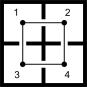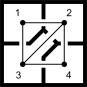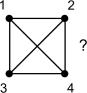4 rooms diagonal K4 Figure 23 Four connected rooms

According to K4, each of the four rooms, may be linked by 3 openings mutually among themselves. The left shows the solution with two openings where one may circulate. The corresponding graph (a circuit) has also been drawn. The middle one demonstrates a solution where only two of the four rooms have 3 doors. To solve the complete graph K4, a ‘dual map’ must be drawn. To do that, K4 is made isomorphically planar, and the planes are interpreted as ‘dual nodes’. The outer area of the graph is involved as a large, encircling dual ‘node’.

These dual nodes (white in the drawing below) should be connected in such a way by dual branches (dotted lines) that all planar branches will be cut through just once: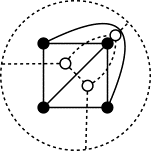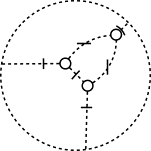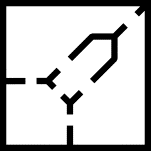Isomorphic planar K4 Dual K4 with doors An architec­tural solution Figure 24 Dual graph

These cuttings through have become ‘doors’ (or windows) in the dual graph. The dual lines are ‘walls’ of a dimension-less  blue-print and the dual points are constructive inter-connections. If this prototypical blue-print is regarded as ‘elastic’, it can be transfigured in an isomorphic way into a design, by giving the surfaces at random forms and shapes. Type K4 is usual for bathrooms and museums.

From a fundamental point of view, no solution exists in flatland for 5 rooms, each sharing one door with all other rooms (K5): no planar graph could be drawn of K5.

By regarding each space firstly as a node between other spaces, the design possibility of programmatic requirements can be verified along the lines of graph theory. Suppose, that the programme of relations between rooms in a dwelling results in the following scheme: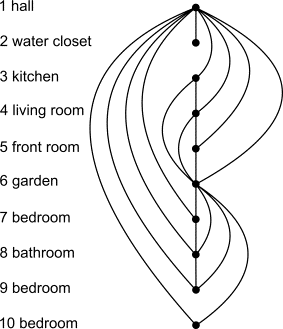Figure 25 Possible relations between rooms

This graph demonstrates that a third bedroom (10) can not be connected to the bathroom if it is already connected to the hall (1) and the garden (6). When the requirement that all bedrooms should give to the garden is skipped (the connection 9-6), a solution exists in which all bedrooms give access to the bathroom.

At 10 rooms, combinatorially speaking 10!/2!8! = 45 relations exist (K10). They can never be established directly (made planar):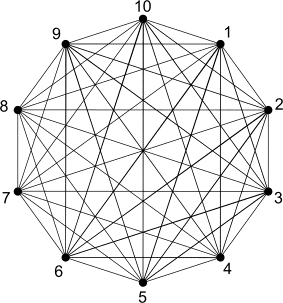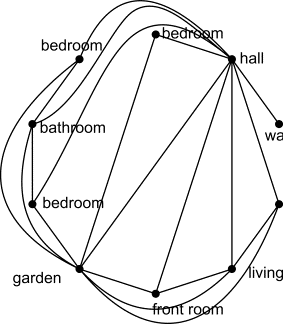K10 Planar selection Figure 26 Planar selection of possible relations

Yet, the selection of the relational scheme can be made planar, therefore, it has a solution. With their high valence (number of doors), the hall (1) and the garden (6) are crucial. If these nodes are removed, a ‘non-conjunctive’ graph originates. Following that, it may be decided to give the rooms 6 to 10 a separate floor; or even its own location. If a node alone allows this freedom, it is termed a ‘separational node’ The minimal number of nodes n that can be taken away with the incident branches in order to make them non-conjunctive makes the graph ‘n-conjunctive’. It is an important measure of cohesion in a system. In the figure following possible realisations are given by drawing the dual graph: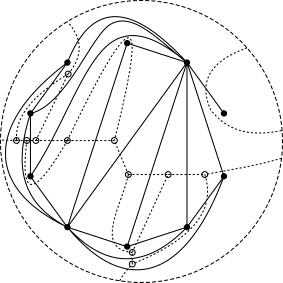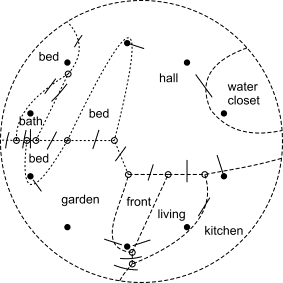Planar + dual Dual+doors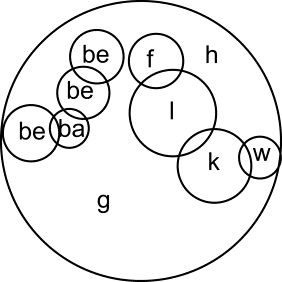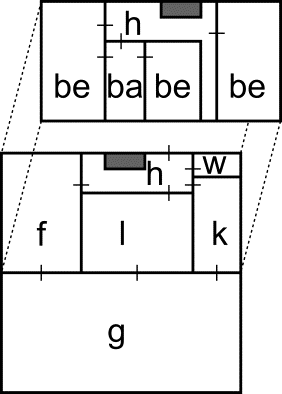Solution 2 wings Solution 2 levels Figure 27 Different solutions of the same dual graph

A map of the national roads of our country is an example of a network. Each branch is denoting a stretch of road, each node a crossing or roundabout. By supplying branches with a length the user can determine quite simply the distance between his point of departure and that of his destination. If the traffic streams across the network and their intensities are known it is easy to determine the traffic load on each branch. A network like this may also represent the traffic deployment within a building, where the branches stand for corridors, stairs and elevators. Then it can be determined, for instance, how many students change places in between classes in the building. This gives a basis for deciding on dimensioning the corridors e. t. q.

The organisational structure of an enterprise may also be depicted in a network. If this structure should be expressed in the building at the design of a new office, this network may form a point of departure; one may derive from it which departments are directly linked to one another with the wish to realise this physically in the new building.

By the same token networks enable the structure of a building or an area, without describing the whole building or the whole area.

A graph with a weight (stream, flow) of any type (time, distance), on its branches, is called a network. If this flow displays a direction as well, the network is termed a directed graph. A path is a sequence connecting branches with a direction and a flow. A network is a cyclical network, if a node connects with itself via a path. The length of the path is determined by the sum of the weight of the paths concerned. The length of the shortest path between two nodes may be determined by following, step by step, a sequence of calculating rules, the shortest-path algorithm.

An algorithm in this vein exists for the longest path, the 'critical-path method'. This method is used in 'network planning' in order to determine, within a project, the earliest and latest moments of starting and finalising each and any activity of the project; and, consequently the minimal duration of the project. In this, the nodes represent the activities, the branches the relation between the activities. The start of an activity (successor) is determined by the time of finalisation of all preceding activities (predecessors). With regard to the network planning, it is feasible to monitor the progress of (complex) projects; as well as to survey the consequences of retardation of activities. However, the problems caused by retardation are not able to be solved. In order to achieve such a solution one has to employ other techniques; like linear programming (LP).

### 24.15    Probability

An event is a subset of results A from a much larger set of possible results W (a set much larger) that might have yielded different results as well. The chance of an event of A well-defined instances taking on ''what had been possible'' W is - expressed in numbers - A/W. Often it is not easy to get an idea of what would have been possible; certainly when W has sub-sets dependent on A, for the event may influence the remaining possibilities.

Say, that the number of possibilities for filling in a surface with 100 buildings from 0 to 9 floors is 10010. Two of these possible events have been drawn below: an example of ‘wild’ and one of ordered housing. The chance that with a maximal height of 9 floors one of the two will be realised exactly is 2 in 10010 (summation rule).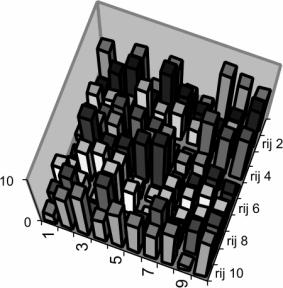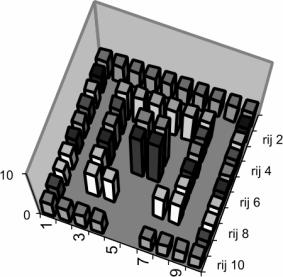Figure 28 Wild and ordered housing

The wild housing leaves the elevation of a building completely to contingency. As a consequence in 100 buildings the average elevation is approximating the middle of 4,5 between 0 and 9. The average of 2.2 floors of ordered housing is deviating more from the mean than the one of wild housing and, therefore, less ‘probable’. In the matrix below the elevations from the drawing of the wild housing have been rendered.

 columns rows 1 2 3 4 5 6 7 8 9 10 mean per row 1 5 0 6 1 6 2 1 8 7 6 4,2 2 1 5 7 2 8 5 3 1